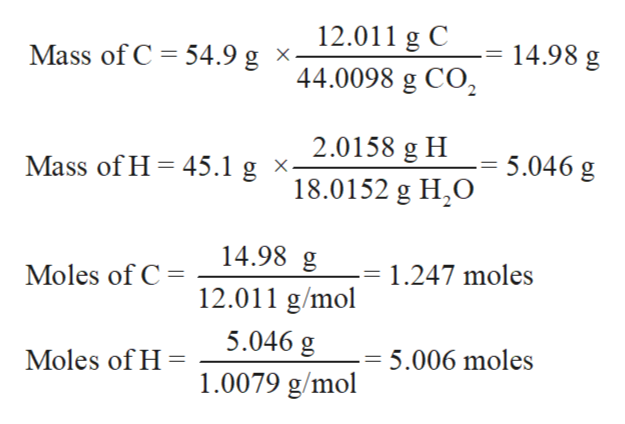# Combustion of an unknown compound containing only carbon and hydrogen produces 54.9 g of CO₂ and 45.1 g of H₂O. What is the empirical formula of the compound?

Question
176 views

Combustion of an unknown compound containing only carbon and hydrogen produces 54.9 g of CO₂ and 45.1 g of H₂O. What is the empirical formula of the compound?

check_circle

Step 1

The empirical formula is the chemical formula of any compound that represents the proportions of each elements that is present in the given compound. It does not show the actual number of element as well as cannot give any information about arrangement of atoms in the formula of compound.

Step 2

Mass of CO2 = 54.9 g

Mass of H2O = 45.1 g

Determine the grams of C and H and convert grams of C and H to their respective amount of moles.

Molar mass of C = 12.01 g/mol

Molar mass of H  =1.0079 g/molhelp_outlineImage Transcriptionclose12.011 g C Mass of C 14.98 g 54.9 g x- 44.0098 g CO2 2.0158 g H Mass of H 45.1 g x. 5.046 g 18.0152 g H2O 14.98 g Moles of C 1.247 moles 12.011 g/mol 5.046 g Moles of H 5.006 moles 1.0079 g/mol fullscreen
Step 3

Divide by least number of moles to get the whol...

### Want to see the full answer?

See Solution

#### Want to see this answer and more?

Solutions are written by subject experts who are available 24/7. Questions are typically answered within 1 hour.*

See Solution
*Response times may vary by subject and question.
Tagged in

### Chemistry